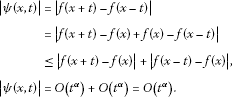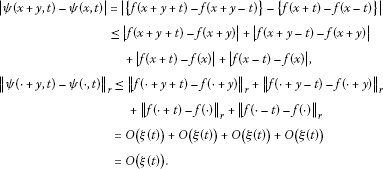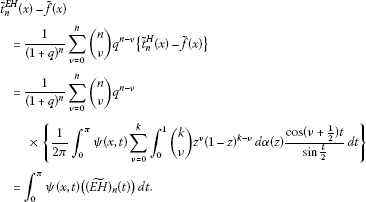# Euler-Hausdorff matrix summability operator and trigonometric approximation of the conjugate of a function belonging to the generalized Lipschitz class

## Abstract

In this paper, three new estimates for the degree of approximation of a function $\stackrel{˜}{f}$, the conjugate of a function f belonging to classes Lipα and $Lip\left(\xi ,r\right)$, $r\ge 1$, by ${E}^{\left(q\right)}\cdot {\mathrm{\Delta }}_{H}$ summability operator of conjugate series of the Fourier series have been determined.

MSC:42A24, 41A25, 42B05, 42B08.

## 1 Introduction

The degree of approximation of the function $f\in Lip\alpha$ and $Lip\left(\alpha ,r\right)$, $r\ge 1$, classes have been determined by several investigators like Alexits , Sahney and Goel , Chandra , Qureshi [4, 5] and Qureshi and Nema . After quite a good amount of work on the degree of approximation of functions by different summability means of its Fourier series, Lal and Singh  established the degree of approximation of conjugates of $Lip\left(\alpha ,r\right)$ functions by $\left(C,1\right)\left(E,1\right)$ product means of conjugate series of a Fourier Series in the following form.

Theorem 1.1 If $f:\mathbb{R}\to \mathbb{R}$ is a 2π-periodic and $Lip\left(\alpha ,r\right)$ function, then the degree of approximation of its conjugate function $\stackrel{˜}{f}$ by the $\left(C,1\right)\left(E,1\right)$ product means of conjugate series of the Fourier series of f satisfies

${M}_{n}\left(\stackrel{˜}{f}\right)=min{\parallel {\left(CE\right)}_{n}^{1}-\stackrel{˜}{f}\parallel }_{r}=O\left(\frac{1}{{\left(n+1\right)}^{\alpha -1/p}}\right),$

where ${\left(CE\right)}_{n}^{1}=\frac{1}{n+1}{\sum }_{k=0}^{n}\frac{1}{{2}^{k}}{\sum }_{j=0}^{k}\left(\genfrac{}{}{0}{}{k}{j}\right){s}_{j}$ is $\left(C,1\right)\left(E,1\right)$ means of conjugate series of the Fourier series.

Recently Nigam and Sharma have obtained the degree of approximation by the Karmata summability method  and also by $\left(C,1\right)\left(E,q\right)$ means of its Fourier series  as follows.

Theorem 1.2 If a function f is 2π-periodic, Lebesgue integrable on $\left[0,2\pi \right]$ and belongs to $Lip\left(\xi \left(t\right),r\right)$ class, then its degree of approximation by $\left(C,1\right)\left(E,q\right)$ summability means of its Fourier series is given by

${\parallel {C}_{n}^{1}{E}_{n}^{q}-f\parallel }_{r}=O\left[{\left(n+1\right)}^{\frac{1}{r}}\xi \left(\frac{1}{n+1}\right)\right]$

provided $\xi \left(t\right)$ satisfies the following conditions:

${\left\{{\int }_{0}^{\frac{1}{n+1}}{\left(\frac{t|\varphi \left(t\right)|}{\xi \left(t\right)}\right)}^{r}\phantom{\rule{0.2em}{0ex}}dt\right\}}^{\frac{1}{r}}=o\left(\frac{1}{n+1}\right)$
(1.1)

and

${\left\{{\int }_{\frac{1}{n+1}}^{\pi }{\left(\frac{{t}^{-\delta }|\varphi \left(t\right)|}{\xi \left(t\right)}\right)}^{r}\phantom{\rule{0.2em}{0ex}}dt\right\}}^{\frac{1}{r}}=O\left({\left(n+1\right)}^{\delta }\right),$
(1.2)

where δ is an arbitrary positive number such that $s\left(1-\delta \right)-1>0$, $\frac{1}{r}+\frac{1}{s}=1$, $1\le r\le \mathrm{\infty }$, conditions (1.1) and (1.2) hold uniformly in x and ${C}_{n}^{1}{E}_{n}^{q}$ is $\left(C,1\right)\left(E,q\right)$ means of the Fourier series.

Working in a slightly different direction, in this paper, the degree of approximation of a function $\stackrel{˜}{f}$, the conjugate of a function f belonging to classes Lipα and $Lip\left(\xi ,r\right)$, $r\ge 1$, by the product summability operator ${E}^{\left(q\right)}\cdot {\mathrm{\Delta }}_{H}$ of conjugate series of the Fourier series has been established. The results are new, sharper and better than previously known results. Furthermore, some interesting particular estimates have been also derived from the main theorems.

## 2 Preliminaries

Let $f\left(x\right)$ be a 2π-periodic function, Lebesgue integrable on $\left[0,2\pi \right]$ and belong to $Lip\left(\xi ,r\right)$ class. The Fourier series of $f\left(x\right)$ is given by

$f\left(x\right)=\frac{1}{2}{a}_{0}+\sum _{n=1}^{\mathrm{\infty }}\left({a}_{n}cosnx+{b}_{n}sinnx\right)=\frac{1}{2}{a}_{0}+\sum _{n=1}^{\mathrm{\infty }}{A}_{n}\left(x\right)$
(2.1)

with n th partial sum ${s}_{n}\left(f;x\right)$.

The series

$\sum _{n=1}^{\mathrm{\infty }}\left({a}_{n}sinnx-{b}_{n}cosnx\right)=-\sum _{n=1}^{\mathrm{\infty }}{B}_{n}\left(x\right)$
(2.2)

is called ‘conjugate series’ of the Fourier series (2.1) with n th partial sum ${\stackrel{˜}{s}}_{n}\left(f;x\right)$.

A Housdorff matrix is a lower triangular matrix with entries

${h}_{n,k}=\left(\genfrac{}{}{0}{}{n}{k}\right){\mathrm{\Delta }}^{n-k}{\mu }_{k},$

where Δ is the forward difference operator defined by $\mathrm{\Delta }{\mu }_{k}={\mu }_{k}-{\mu }_{k+1}$ and $\mathrm{\Delta }\left({\mathrm{\Delta }}^{n}{\mu }_{k}\right)={\mathrm{\Delta }}^{n+1}{\mu }_{k}$.

Let ${\sum }_{n=0}^{\mathrm{\infty }}{u}_{n}$ be an infinite series with n th partial sum ${s}_{n}={\sum }_{k=0}^{n}{u}_{k}$.

If ${t}_{n}^{H}={\sum }_{k=0}^{n}{h}_{n,k}{s}_{k}\to s$ as $n\to \mathrm{\infty }$, ${\sum }_{n=0}^{\mathrm{\infty }}{u}_{n}$ is said to be summable to the sum s by the Hausdorff matrix summability method (${\mathrm{\Delta }}_{H}$ means) (Boos and Cass ).

The Hausdorff matrix H is regular, i.e., H preserves the limit of each convergent sequence if and only if

${\int }_{0}^{1}|d\left(\alpha \left(z\right)\right)|<\mathrm{\infty },$

where the mass function $\alpha \in BV\left[0,1\right]$, $\alpha \left(0+\right)=\alpha \left(0\right)=0$, and $\alpha \left(1\right)=1$. In this case, the ${\mu }_{n}$ have the representation

${\mu }_{n}={\int }_{0}^{1}{z}^{n}\phantom{\rule{0.2em}{0ex}}d\alpha \left(z\right).$

Let ${E}_{n}^{\left(q\right)}=\frac{1}{{\left(1+q\right)}^{n}}{\sum }_{k=0}^{n}\left(\genfrac{}{}{0}{}{n}{k}\right){q}^{n-k}{s}_{k}$, $q>0$.

If ${E}_{n}^{\left(q\right)}\to s$ as $n\to \mathrm{\infty }$, ${\sum }_{n=0}^{\mathrm{\infty }}{u}_{n}$ is said to be summable to s by the Euler method $\left(E,q\right)$ (Hardy , p.180).

The $\left(E,q\right)$ transform of the ${t}_{n}^{H}$ transform defines the ${E}^{\left(q\right)}\cdot {\mathrm{\Delta }}_{H}$ transform of $\left({s}_{n}\right)$. It is denoted by ${t}_{n}^{EH}$. Thus,

${t}_{n}^{EH}=\frac{1}{{\left(1+q\right)}^{n}}\sum _{k=0}^{n}\left(\genfrac{}{}{0}{}{n}{k}\right){q}^{n-k}{t}_{k}^{{\mathrm{\Delta }}_{H}}=\frac{1}{{\left(1+q\right)}^{n}}\sum _{k=0}^{n}\left(\genfrac{}{}{0}{}{n}{k}\right){q}^{n-k}\sum _{\nu =0}^{k}{h}_{k,\nu }{s}_{\nu }.$

If ${t}_{n}^{EH}\to s$ as $n\to \mathrm{\infty }$, $\sum {u}_{n}$ is said to be summable to s by the Euler-Hausdorff matrix summability means, i.e., ${E}^{\left(q\right)}\cdot {\mathrm{\Delta }}_{H}$ means.

Thus, if the method of summability $\left(E,q\right)$ is superimposed on the ${t}_{n}^{H}$ method, another new method of summability ${E}^{\left(q\right)}\cdot {\mathrm{\Delta }}_{H}$ is obtained.

The important particular cases of ${E}^{q}\cdot {\mathrm{\Delta }}_{H}$ means are as follows:

1. 1.

$\left(E,q\right)\left(C,\delta \right)$ if $\alpha \left(z\right)={\prod }_{k=1}^{\delta }{z}^{k}$, $\delta \ge 1$,

2. 2.

$\left(E,1\right)\left(C,\delta \right)$ if $q=1$ and $\alpha \left(z\right)={\prod }_{k=1}^{\delta }{z}^{k}$, $\delta \ge 1$,

3. 3.

$\left(E,q\right)\left(C,1\right)$ if $\alpha \left(z\right)=z$,

4. 4.

$\left(E,1\right)\left(C,1\right)$ if $q=1$ and $\alpha \left(z\right)=z$.

Let ${\parallel \phantom{\rule{0.25em}{0ex}}\parallel }_{\mathrm{\infty }}$ of a function $f:\left[0,2\pi \right]\to \mathbb{R}$ be defined by

${\parallel f\parallel }_{\mathrm{\infty }}=ess\underset{0\le x\le 2\pi }{sup}|f\left(x\right)|$

and the degree of approximation of a function $f:\left[0,2\pi \right]\to \mathbb{R}$ by a trigonometric polynomial of order n, ${t}_{n}=\frac{1}{2}{a}_{0}+{\sum }_{\nu =1}^{n}\left({a}_{\nu }cos\nu x+{b}_{\nu }sin\nu x\right)$, under esssup norm ${\parallel \phantom{\rule{0.25em}{0ex}}\parallel }_{\mathrm{\infty }}$ be defined by (Zygmund , p.114)

${\parallel {t}_{n}-f\parallel }_{\mathrm{\infty }}=ess\underset{0\le x\le 2\pi }{sup}|{t}_{n}\left(x\right)-f\left(x\right)|.$

We define the norm ${L}^{r}={\parallel \phantom{\rule{0.25em}{0ex}}\parallel }_{r}$ by ${\parallel f\parallel }_{r}={\left\{\frac{1}{2\pi }{\int }_{0}^{2\pi }|f\left(x\right){|}^{r}\phantom{\rule{0.2em}{0ex}}dr\right\}}^{1/r}$, $r\ge 1$, and let the degree of trigonometric approximation ${E}_{n}\left(f\right)$ be given by

${E}_{n}\left(f\right)=\underset{{t}_{n}}{min}{\parallel f-{t}_{n}\parallel }_{r}.$

Define the norm ${\parallel \phantom{\rule{0.25em}{0ex}}\parallel }_{r}^{\left(\xi \right)}$ on class ${L}_{\left(\xi \right)}^{r}$ of functions by

${\parallel f\parallel }_{r}^{\left(\xi \right)}=\underset{t\ne 0}{sup}\frac{{\parallel f\left(\cdot +t\right)-f\left(\cdot \right)\parallel }_{r}}{\xi \left(|t|\right)}.$

The degree of approximation ${E}_{n}\left(f;{L}_{r}^{\left(\xi \right)}\right)$ of a function f belonging to $Lip\left(\xi ,r\right)$ class under the norm ${\parallel \phantom{\rule{0.25em}{0ex}}\parallel }_{r}^{\left(u\right)}$ is given by

${E}_{n}\left(f;{L}_{\left(\xi \right)}^{r}\right)={\parallel {t}_{n}-f\parallel }_{r}^{\left(u\right)}.$

A function $f\in Lip\alpha$ if $|f\left(x+t\right)-f\left(x\right)|=O\left(|t{|}^{\alpha }\right)$ for $0<\alpha \le 1$ (Titchmarsh , p.426).

$f\in Lip\left(\alpha ,r\right)$ if ${\left\{{\int }_{0}^{2\pi }|f\left(x+t\right)-f\left(x\right){|}^{r}\phantom{\rule{0.2em}{0ex}}dx\right\}}^{\frac{1}{r}}=O\left(|t{|}^{\alpha }\right)$ for $0<\alpha \le 1$, $r\ge 1$ (def. 5.38 of McFadden ).

$f\in Lip\left(\xi ,r\right)$ if ${\left\{{\int }_{0}^{2\pi }|f\left(x+t\right)-f\left(x\right){|}^{r}\phantom{\rule{0.2em}{0ex}}dx\right\}}^{1/r}=O\left(\xi \left(t\right)\right)$ ($r\ge 1$), where ξ is a modulus of continuity, that is, ξ is a non-negative, non-decreasing, continuous function with the properties $\xi \left(0\right)=0$, $\xi \left({t}_{1}+{t}_{2}\right)\le \xi \left({t}_{1}\right)+\xi \left({t}_{2}\right)$ (Khan ).

If $\xi \left(t\right)={t}^{\alpha }$, $Lip\left(\xi ,r\right)$ class coincides with the class $Lip\left(\alpha ,r\right)$, and if $r\to \mathrm{\infty }$, then $Lip\left(\alpha ,r\right)$ reduces to Lipα class. Thus, it is obvious that $Lip\alpha \subseteq Lip\left(\alpha ,r\right)\subseteq Lip\left(\xi ,r\right)$.

Let u be a modulus of continuity such that $\frac{\xi \left(t\right)}{u\left(t\right)}$ is positive, non-decreasing.

Then ${\parallel f\parallel }_{r}^{\left(u\right)}\le max\left(1,\frac{\xi \left(2\pi \right)}{u\left(\pi \right)}\right){\parallel f\parallel }_{r}^{\left(\xi \right)}$. Thus, ${L}_{\left(\xi \right)}^{r}\subseteq {L}_{\left(u\right)}^{r}\subseteq {L}^{r}$.

We write $\psi \left(x,t\right)=f\left(x+t\right)-f\left(x-t\right)$, $\stackrel{˜}{f}\left(x\right)=-\frac{1}{2\pi }{\int }_{0}^{\pi }\psi \left(x,t\right)cot\frac{t}{2}\phantom{\rule{0.2em}{0ex}}dt$,

${\left(\stackrel{˜}{EH}\right)}_{n}\left(t\right)=\frac{1}{2\pi {\left(1+q\right)}^{n}}\sum _{k=0}^{n}\left(\genfrac{}{}{0}{}{n}{k}\right){q}^{n-k}\sum _{\nu =0}^{k}{\int }_{0}^{1}\left(\genfrac{}{}{0}{}{k}{\nu }\right){z}^{\nu }{\left(1-z\right)}^{k-\nu }\phantom{\rule{0.2em}{0ex}}d\alpha \left(z\right)\frac{cos\left(\nu +\frac{1}{2}\right)t}{sin\frac{t}{2}}.$

## 3 Results

In this paper, three new estimates for the degree of approximation of a function $\stackrel{˜}{f}$, the conjugate of a function f belonging to the classes Lipα and $Lip\left(\xi ,r\right)$ ($r\ge 1$) by ${E}^{\left(q\right)}\cdot {\mathrm{\Delta }}_{H}$ summability operator have been determined in the following form.

Theorem 3.1 If $f:\left[0,2\pi \right]\to \mathbb{R}$ is 2π-periodic, Lebesgue integrable on $\left[0,2\pi \right]$ and belongs to the class Lipα, then the degree of approximation of $\stackrel{˜}{f}$, the conjugate of f, by ${E}^{\left(q\right)}\cdot {\mathrm{\Delta }}_{H}$ means

${t}_{n}^{EH}=\frac{1}{{\left(1+q\right)}^{n}}\sum _{k=0}^{n}\left(\genfrac{}{}{0}{}{n}{k}\right){q}^{n-k}\sum _{\nu =0}^{k}{h}_{k,\nu }{s}_{\nu }$

of conjugate series (2.2) of its Fourier series (2.1) satisfies, for $n=0,1,2,3,\dots$ ,

${\parallel {\stackrel{˜}{t}}_{n}^{EH}-\stackrel{˜}{f}\parallel }_{\mathrm{\infty }}=ess\underset{0\le x\le 2\pi }{sup}\left\{{\stackrel{˜}{t}}_{n}^{EH}\left(x\right)-\stackrel{˜}{f}\left(x\right)\right\}=\left\{\begin{array}{cc}O\left({\left(n+1\right)}^{-\alpha }\right),\hfill & 0<\alpha <1,\hfill \\ O\left(\frac{log\left(n+1\right)\pi }{\left(n+1\right)}\right),\hfill & \alpha =1.\hfill \end{array}$
(3.1)

Theorem 3.2 Let $\xi \left(t\right)$ be the modulus of continuity such that

${\int }_{0}^{v}\frac{\xi \left(t\right)}{t}\phantom{\rule{0.2em}{0ex}}dt=O\left(\xi \left(v\right)\right),\phantom{\rule{1em}{0ex}}0
(3.2)

If $f:\left[0,2\pi \right]\to \mathbb{R}$ is 2π-periodic, Lebesgue integrable on $\left[0,2\pi \right]$ and belongs to the class $Lip\left(\xi ,r\right)$ ($r\ge 1$), then the degree of approximation of $\stackrel{˜}{f}$, the conjugate of f, by ${E}^{\left(q\right)}\cdot {\mathrm{\Delta }}_{H}$ means ${\stackrel{˜}{t}}_{n}^{EH}$ of conjugate series (2.2) of its Fourier series (2.1) is given by

(3.3)

Theorem 3.3 The degree of approximation ${E}_{n}\left(f;{L}_{r}^{\left(\xi \right)}\right)$ of a function $\stackrel{˜}{f}$, the conjugate of a function f belonging to $Lip\left(\xi ,r\right)$ class, by ${\stackrel{˜}{t}}_{n}^{EH}$ means of conjugate series (2.2) is given by

${\parallel {\stackrel{˜}{t}}_{n}^{EH}-\stackrel{˜}{f}\parallel }_{r}^{\left(u\right)}=O\left(\frac{1}{n+1}{\int }_{\frac{1}{n+1}}^{\pi }\frac{\xi \left(t\right)}{{t}^{2}u\left(t\right)}\phantom{\rule{0.2em}{0ex}}dt\right),$
(3.4)

where $\xi \left(t\right)$ and $u\left(t\right)$ are the modulus of continuity such that

${\int }_{0}^{v}\frac{\xi \left(t\right)}{tu\left(t\right)}\phantom{\rule{0.2em}{0ex}}dt=O\left(\frac{\xi \left(v\right)}{u\left(v\right)}\right),\phantom{\rule{1em}{0ex}}0
(3.5)

## 4 Lemmas

For the proof of our theorems, the following lemmas are required.

Lemma 4.1 ${\left(\stackrel{˜}{EH}\right)}_{n}\left(t\right)=O\left(\frac{1}{t}\right)$ for $0.

Proof For $0, $|cost|\le 1$, $sin\left(t/2\right)\ge \left(t/\pi \right)$ and ${sup}_{0\le z\le 1}|{\alpha }^{\prime }\left(z\right)|=N$, we have

$\begin{array}{rcl}|{\left(\stackrel{˜}{EH}\right)}_{n}\left(t\right)|& =& |\frac{1}{2\pi {\left(1+q\right)}^{n}}\sum _{k=0}^{n}\left(\genfrac{}{}{0}{}{n}{k}\right){q}^{n-k}\sum _{\nu =0}^{k}{\int }_{0}^{1}\left(\genfrac{}{}{0}{}{k}{\nu }\right){z}^{\nu }{\left(1-z\right)}^{k-\nu }\phantom{\rule{0.2em}{0ex}}d\alpha \left(z\right)\frac{cos\left(\nu +\frac{1}{2}\right)t}{sin\frac{t}{2}}|\\ \le & \frac{1}{2\pi {\left(1+q\right)}^{n}}\sum _{k=0}^{n}\left(\genfrac{}{}{0}{}{n}{k}\right){q}^{n-k}\sum _{\nu =0}^{k}{\int }_{0}^{1}\left(\genfrac{}{}{0}{}{k}{\nu }\right){z}^{\nu }{\left(1-z\right)}^{k-\nu }\phantom{\rule{0.2em}{0ex}}d\alpha \left(z\right)\frac{|cos\left(\nu +\frac{1}{2}\right)t|}{|sin\frac{t}{2}|}\\ \le & \frac{1}{2\pi {\left(1+q\right)}^{n}}\sum _{k=0}^{n}\left(\genfrac{}{}{0}{}{n}{k}\right){q}^{n-k}\sum _{\nu =0}^{k}{\int }_{0}^{1}\left(\genfrac{}{}{0}{}{k}{\nu }\right){z}^{\nu }{\left(1-z\right)}^{k-\nu }\phantom{\rule{0.2em}{0ex}}d\alpha \left(z\right)\frac{1}{t/\pi }\\ \le & \frac{N}{2t{\left(1+q\right)}^{n}}\sum _{k=0}^{n}\left(\genfrac{}{}{0}{}{n}{k}\right){q}^{n-k}{\int }_{0}^{1}\sum _{\nu =0}^{k}\left(\genfrac{}{}{0}{}{k}{\nu }\right){z}^{\nu }{\left(1-z\right)}^{k-\nu }\phantom{\rule{0.2em}{0ex}}dz\\ =& \frac{N}{2t{\left(1+q\right)}^{n}}\sum _{k=0}^{n}\left(\genfrac{}{}{0}{}{n}{k}\right){q}^{n-k}{\int }_{0}^{1}{\left(z+1-z\right)}^{k}\phantom{\rule{0.2em}{0ex}}dz\\ =& \frac{N}{2t{\left(1+q\right)}^{n}}\sum _{k=0}^{n}\left(\genfrac{}{}{0}{}{n}{k}\right){q}^{n-k}=O\left(\frac{1}{t}\right).\end{array}$

□

Lemma 4.2 ${\left(\stackrel{˜}{EH}\right)}_{n}\left(t\right)=O\left(\frac{1}{\left(n+1\right){t}^{2}}\right)$ for $\frac{1}{n+1}.

Proof For $\frac{1}{n+1}, $sin\left(n+1\right)t\le 1$, $sin\left(t/2\right)\ge \left(t/\pi \right)$ and ${sup}_{0\le z\le 1}|{\alpha }^{\prime }\left(z\right)|=N$

$\begin{array}{c}\sum _{k=0}^{n}{\int }_{0}^{1}\left(\genfrac{}{}{0}{}{n}{k}\right){z}^{k}{\left(1-z\right)}^{n-k}{e}^{i\left(k+\frac{1}{2}\right)t}\phantom{\rule{0.2em}{0ex}}d\alpha \left(z\right)\hfill \\ \phantom{\rule{1em}{0ex}}={\int }_{0}^{1}{e}^{it/2}\sum _{k=0}^{n}\left(\genfrac{}{}{0}{}{n}{k}\right){z}^{k}{\left(1-z\right)}^{n-k}{e}^{ikt}\phantom{\rule{0.2em}{0ex}}d\alpha \left(z\right)\hfill \\ \phantom{\rule{1em}{0ex}}={\int }_{0}^{1}{e}^{it/2}{\left(1-z\right)}^{n}\sum _{k=0}^{n}\left(\genfrac{}{}{0}{}{n}{k}\right){\left(\frac{z}{1-z}\right)}^{k}{e}^{ikt}\phantom{\rule{0.2em}{0ex}}d\alpha \left(z\right)\hfill \\ \phantom{\rule{1em}{0ex}}={\int }_{0}^{1}{e}^{it/2}{\left(1-z+z{e}^{it}\right)}^{n}\phantom{\rule{0.2em}{0ex}}d\alpha \left(z\right)\hfill \\ \phantom{\rule{1em}{0ex}}\le N{\int }_{0}^{1}{e}^{it/2}{\left(1-z+z{e}^{it}\right)}^{n}\phantom{\rule{0.2em}{0ex}}dz\hfill \\ \phantom{\rule{1em}{0ex}}=\frac{N{e}^{it/2}}{\left(n+1\right)}\left(\frac{{e}^{i\left(n+1\right)t}-1}{{e}^{it}-1}\right)\hfill \\ \phantom{\rule{1em}{0ex}}=\frac{N}{\left(n+1\right)}\left(\frac{{e}^{i\left(n+1\right)t}-1}{2isin\frac{t}{2}}\right)\hfill \\ \phantom{\rule{1em}{0ex}}=\frac{N}{\left(n+1\right)}\left(\frac{\left(cos\left(n+1\right)t-1\right)+isin\left(n+1\right)t}{2isin\frac{t}{2}}\right).\hfill \end{array}$

Equating real parts from both sides, we get

$\begin{array}{rcl}\sum _{k=0}^{n}{\int }_{0}^{1}\left(\genfrac{}{}{0}{}{n}{k}\right){z}^{k}{\left(1-z\right)}^{n-k}cos\left(k+\frac{1}{2}\right)\phantom{\rule{0.2em}{0ex}}d\alpha \left(z\right)& =& \frac{N}{\left(n+1\right)}\left(\frac{sin\left(n+1\right)t}{2sin\frac{t}{2}}\right)\\ =& O\left(\frac{1}{\left(n+1\right)t}\right).\end{array}$

Therefore, using ${\sum }_{\nu =0}^{n}\left(\genfrac{}{}{0}{}{n}{\nu }\right)\frac{{q}^{n-\nu }}{\nu +1}=O\left(\frac{{\left(1+q\right)}^{n}}{n+1}\right)$, we get

$\begin{array}{rcl}{\left(\stackrel{˜}{EH}\right)}_{n}\left(t\right)& =& \frac{1}{2\pi {\left(1+q\right)}^{n}}\sum _{k=0}^{n}\left(\genfrac{}{}{0}{}{n}{k}\right){q}^{n-k}\sum _{\nu =0}^{k}{\int }_{0}^{1}\left(\genfrac{}{}{0}{}{k}{\nu }\right){z}^{\nu }{\left(1-z\right)}^{k-\nu }\phantom{\rule{0.2em}{0ex}}d\alpha \left(z\right)\frac{cos\left(\nu +\frac{1}{2}\right)t}{sin\frac{t}{2}}\\ \le & \frac{1}{2t{\left(1+q\right)}^{n}}\sum _{k=0}^{n}\left(\genfrac{}{}{0}{}{n}{k}\right){q}^{n-k}\sum _{\nu =0}^{k}{\int }_{0}^{1}\left(\genfrac{}{}{0}{}{k}{\nu }\right){z}^{\nu }{\left(1-z\right)}^{k-\nu }\phantom{\rule{0.2em}{0ex}}d\alpha \left(z\right)cos\left(\nu +\frac{1}{2}\right)t\\ =& \frac{1}{2t{\left(1+q\right)}^{n}}\sum _{k=0}^{n}\left(\genfrac{}{}{0}{}{n}{k}\right){q}^{n-k}\left\{O\left(\frac{1}{\left(k+1\right)t}\right)\right\}\\ =& O\left(\frac{1}{2{t}^{2}{\left(1+q\right)}^{n}}\sum _{k=0}^{n}\left(\genfrac{}{}{0}{}{n}{k}\right)\frac{{q}^{n-k}}{k+1}\right)=O\left(\frac{1}{\left(n+1\right){t}^{2}}\right).\end{array}$

□

Lemma 4.3 If $f\in Lip\alpha$, then

$|\psi \left(x,t\right)|=O\left({t}^{\alpha }\right).$

Proof

Clearly,□

Lemma 4.4 If $f\in Lip\left(\xi ,r\right)$, $r\ge 1$, then

${\left[{\int }_{0}^{2\pi }|\psi \left(x,t\right){|}^{r}\phantom{\rule{0.2em}{0ex}}dx\right]}^{1/r}=O\left(\xi \left(t\right)\right).$

Proof

Using Lemma 4.3 and Minkowski’s inequality, we have□

Lemma 4.5

${\parallel \psi \left(\cdot +y,t\right)-\psi \left(\cdot ,t\right)\parallel }_{r}=O\left(u\left(|y|\right)\frac{\xi \left(t\right)}{u\left(t\right)}\right).$

Proof

$\begin{array}{rcl}|\psi \left(x+y,t\right)-\psi \left(x,t\right)|& =& |\left\{f\left(x+y+t\right)-f\left(x+y-t\right)\right\}-\left\{f\left(x+t\right)-f\left(x-t\right)\right\}|\\ \le & |f\left(x+y+t\right)-f\left(x+t\right)|+|f\left(x+y-t\right)-f\left(x-t\right)|.\end{array}$

Applying Minkowski’s inequality, we have

$\begin{array}{rcl}{\parallel \psi \left(\cdot +y,t\right)-\psi \left(\cdot ,t\right)\parallel }_{r}& \le & {\parallel f\left(\cdot +y+t\right)-f\left(\cdot +t\right)\parallel }_{r}+{\parallel f\left(\cdot +y-t\right)-f\left(\cdot +t\right)\parallel }_{r}\\ =& O\left(\xi \left(|y|\right)\right)+O\left(\xi \left(|y|\right)\right)=O\left(\xi \left(|y|\right)\right).\end{array}$
(4.1)

Also, we can write(4.2)

For $t\le |y|$, we obtain

$\begin{array}{rcl}{\parallel \psi \left(\cdot +y,t\right)-\psi \left(\cdot ,t\right)\parallel }_{r}& =& O\left(\xi \left(t\right)\right)\\ =& O\left(u\left(|y|\right)\frac{\xi \left(t\right)}{u\left(t\right)}\right).\end{array}$

Since $\frac{\xi \left(t\right)}{u\left(t\right)}$ is positive, non-decreasing, if $t\ge |y|$, then $\frac{\xi \left(t\right)}{u\left(t\right)}\ge \frac{\xi \left(|y|\right)}{u\left(|y|\right)}$, so that

$\begin{array}{rcl}{\parallel \psi \left(\cdot +y,t\right)-\psi \left(\cdot ,t\right)\parallel }_{r}& =& O\left(\xi \left(|y|\right)\right)\\ =& O\left(u\left(|y|\right)\frac{\xi \left(t\right)}{u\left(t\right)}\right).\end{array}$
(4.3)

□

Lemma 4.6 The inequality

${\left\{\frac{1}{2\pi }{\int }_{0}^{2\pi }|{\int }_{a}^{b}g\left(x,t\right)\phantom{\rule{0.2em}{0ex}}dt{|}^{r}\phantom{\rule{0.2em}{0ex}}dx\right\}}^{1/r}\le {\int }_{a}^{b}{\left\{\frac{1}{2\pi }{\int }_{0}^{2\pi }|g\left(t,x\right){|}^{r}\phantom{\rule{0.2em}{0ex}}dx\right\}}^{1/r}\phantom{\rule{0.2em}{0ex}}dt$

is known as generalized Minkowski’s inequality where the generalization is simply replacing a finite sum by a definite (Lebesgue) integral (see Chui ).

## 5 Proof of Theorem 3.1

The n th partial sum of conjugate series (2.2) is given by

${\stackrel{˜}{s}}_{n}\left(f;x\right)-\stackrel{˜}{f}\left(x\right)=\frac{1}{2\pi }{\int }_{0}^{\pi }\psi \left(x,t\right)\frac{cos\left(n+\frac{1}{2}\right)t}{sin\frac{t}{2}}\phantom{\rule{0.2em}{0ex}}dt.$

Denoting the Hausdorff matrix summability transform of ${\stackrel{˜}{s}}_{n}\left(f;x\right)$ by ${\stackrel{˜}{t}}_{n}^{H}\left(x\right)$, we get

$\begin{array}{rcl}{\stackrel{˜}{t}}_{n}^{H}\left(x\right)-\stackrel{˜}{f}\left(x\right)& =& \sum _{k=0}^{n}{h}_{n,k}\left\{{\stackrel{˜}{s}}_{k}\left(f;x\right)-\stackrel{˜}{f}\left(x\right)\right\}\\ =& \sum _{k=0}^{n}\left(\genfrac{}{}{0}{}{n}{k}\right){\mathrm{\Delta }}^{n-k}{\mu }_{k}\left\{\frac{1}{2\pi }{\int }_{0}^{\pi }\psi \left(x,t\right)\frac{cos\left(k+\frac{1}{2}\right)t}{sin\frac{t}{2}}\phantom{\rule{0.2em}{0ex}}dt\right\}\\ =& \frac{1}{2\pi }{\int }_{0}^{\pi }\psi \left(x,t\right)\sum _{k=0}^{n}\left(\genfrac{}{}{0}{}{n}{k}\right){\mathrm{\Delta }}^{n-k}\left({\int }_{0}^{1}{z}^{k}\phantom{\rule{0.2em}{0ex}}d\alpha \left(z\right)\right)\frac{cos\left(k+\frac{1}{2}\right)t}{sin\frac{t}{2}}\phantom{\rule{0.2em}{0ex}}dt\\ =& \frac{1}{2\pi }{\int }_{0}^{\pi }\psi \left(x,t\right)\sum _{k=0}^{n}{\int }_{0}^{1}\left(\genfrac{}{}{0}{}{n}{k}\right){z}^{k}{\left(1-z\right)}^{n-k}\phantom{\rule{0.2em}{0ex}}d\alpha \left(z\right)\frac{cos\left(k+\frac{1}{2}\right)t}{sin\frac{t}{2}}\phantom{\rule{0.2em}{0ex}}dt.\end{array}$

The $\left(E,q\right)$ transform of ${\stackrel{˜}{t}}_{n}^{H}\left(x\right)$, i.e., the ${E}^{\left(q\right)}\cdot {\mathrm{\Delta }}_{H}$ transform of ${\stackrel{˜}{s}}_{n}\left(f;x\right)$ denoted by ${\stackrel{˜}{t}}_{n}^{EH}\left(x\right)$, is given by(5.1)

Thus,

(5.2)

Applying Lemma 4.1 and Lemma 4.3, we have

$\begin{array}{rcl}{I}_{1}& =& O\left({\int }_{0}^{\frac{1}{\left(n+1\right)}}{t}^{\alpha }{\left(\stackrel{˜}{EH}\right)}_{n}\left(t\right)\phantom{\rule{0.2em}{0ex}}dt\right)\\ =& O\left({\int }_{0}^{\frac{1}{\left(n+1\right)}}{t}^{\alpha -1}\phantom{\rule{0.2em}{0ex}}dt\right)\\ =& O\left({\left(n+1\right)}^{-\alpha }\right).\end{array}$
(5.3)

Now, by Lemma 4.2 and Lemma 4.3, we get

$\begin{array}{rcl}{I}_{2}& =& O\left({\int }_{\frac{1}{\left(n+1\right)}}^{\pi }\frac{{t}^{\alpha }}{\left(n+1\right){t}^{2}}\phantom{\rule{0.2em}{0ex}}dt\right)\\ =& O\left[\frac{1}{\left(n+1\right)}{\int }_{\frac{1}{\left(n+1\right)}}^{\pi }{t}^{\alpha -2}\phantom{\rule{0.2em}{0ex}}dt\right]\\ =& \left\{\begin{array}{cc}O\left[\left(\frac{1}{\left(n+1\right)}\right)\left(\frac{1}{1-\alpha }\right)\left(\frac{1}{{\left(n+1\right)}^{\alpha -1}}-{\pi }^{\alpha -1}\right)\right],\hfill & 0<\alpha <1,\hfill \\ O\left(\frac{log\left(n+1\right)\pi }{\left(n+1\right)}\right),\hfill & \alpha =1\hfill \end{array}\\ =& \left\{\begin{array}{cc}O\left({\left(n+1\right)}^{-\alpha }\right),\hfill & 0<\alpha <1,\hfill \\ O\left(\frac{log\left(n+1\right)\pi }{\left(n+1\right)}\right),\hfill & \alpha =1.\hfill \end{array}\end{array}$
(5.4)

Collecting equations (5.2) to (5.4), the result is

${\parallel {\stackrel{˜}{t}}_{n}^{EH}-\stackrel{˜}{f}\parallel }_{\mathrm{\infty }}=ess\underset{0\le x\le 2\pi }{sup}|{\stackrel{˜}{t}}_{n}^{EH}\left(x\right)-\stackrel{˜}{f}\left(x\right)|=\left\{\begin{array}{cc}O\left({\left(n+1\right)}^{-\alpha }\right),\hfill & 0<\alpha <1,\hfill \\ O\left(\frac{log\left(n+1\right)\pi }{\left(n+1\right)}\right),\hfill & \alpha =1.\hfill \end{array}$

This completes the proof of Theorem 3.1.

## 6 Proof of Theorem 3.2

Following the proof of Theorem 3.1, using equation (5.1), we have

(6.1)

Applying Lemmas 4.1 and 4.4 and condition (3.2), we obtain

$\begin{array}{rcl}{I}_{1}^{\mathrm{\prime }}& =& O\left({\int }_{0}^{\frac{1}{\left(n+1\right)}}\xi \left(t\right){\left(\stackrel{˜}{EH}\right)}_{n}\left(t\right)\phantom{\rule{0.2em}{0ex}}dt\right)\\ =& O\left({\int }_{0}^{\frac{1}{\left(n+1\right)}}\frac{\xi \left(t\right)}{t}\phantom{\rule{0.2em}{0ex}}dt\right)\\ =& O\left(\xi \left(\frac{1}{n+1}\right)\right).\end{array}$
(6.2)

By Lemmas 4.2 and 4.4, we have

$\begin{array}{rcl}{I}_{2}^{\mathrm{\prime }}& =& O\left({\int }_{\frac{1}{\left(n+1\right)}}^{\pi }\frac{\xi \left(t\right)}{\left(n+1\right){t}^{2}}\phantom{\rule{0.2em}{0ex}}dt\right)\\ =& O\left(\frac{1}{\left(n+1\right)}{\int }_{\frac{1}{\left(n+1\right)}}^{\pi }\frac{\xi \left(t\right)}{{t}^{2}}\phantom{\rule{0.2em}{0ex}}dt\right).\end{array}$
(6.3)

Next,

$\begin{array}{rcl}\frac{1}{\left(n+1\right)}{\int }_{\frac{1}{\left(n+1\right)}}^{\pi }\frac{\xi \left(t\right)}{{t}^{2}}\phantom{\rule{0.2em}{0ex}}dt& \ge & \frac{1}{\left(n+1\right)}\xi \left(\frac{1}{\left(n+1\right)}\right){\int }_{\frac{1}{\left(n+1\right)}}^{\pi }\frac{1}{{t}^{2}}\phantom{\rule{0.2em}{0ex}}dt\\ =& \xi \left(\frac{1}{\left(n+1\right)}\right)\left\{1-\frac{1}{\left(n+1\right)\pi }\right\}\ge \frac{1}{2}\xi \left(\frac{1}{\left(n+1\right)}\right).\end{array}$

Then

$\xi \left(\frac{1}{\left(n+1\right)}\right)=O\left(\frac{1}{\left(n+1\right)}{\int }_{\frac{1}{\left(n+1\right)}}^{\pi }\frac{\xi \left(t\right)}{{t}^{2}}\phantom{\rule{0.2em}{0ex}}dt\right).$
(6.4)

Combining equations (6.1) to (6.4), we get

${\parallel {\stackrel{˜}{t}}_{n}^{EH}-\stackrel{˜}{f}\parallel }_{r}=O\left(\frac{1}{\left(n+1\right)}{\int }_{\frac{1}{\left(n+1\right)}}^{\pi }\frac{\xi \left(t\right)}{{t}^{2}}\phantom{\rule{0.2em}{0ex}}dt\right).$

This completes the proof of Theorem 3.2.

## 7 Proof of Theorem 3.3

By equation (5.2) of the proof of Theorem 3.1 and Lemma 4.6, we get(7.1)(7.2)(7.3)

Using Lemma 4.1 and Lemma 4.5 and (3.5), we obtain

$\begin{array}{rcl}{I}_{1}^{\mathrm{\prime }\mathrm{\prime }}& =& {\int }_{0}^{\frac{1}{n+1}}{\parallel \psi \left(\cdot +y,t\right)-\psi \left(\cdot ,t\right)\parallel }_{r}{\left(EH\right)}_{n}\left(t\right)\phantom{\rule{0.2em}{0ex}}dt\\ =& O\left({\int }_{0}^{\frac{1}{n+1}}u\left(|y|\right)\frac{\xi \left(t\right)}{tu\left(t\right)}\phantom{\rule{0.2em}{0ex}}dt\right)=O\left(u\left(|y|\right)\frac{\xi \left(\frac{1}{n+1}\right)}{u\left(\frac{1}{n+1}\right)}\right).\end{array}$
(7.4)

Also, using Lemma 4.2 and Lemma 4.5, we have

(7.5)

By (7.3), (7.4) and (7.5), we have

${\parallel {\stackrel{˜}{l}}_{n}\left(\cdot +y\right)-{\stackrel{˜}{l}}_{n}\left(\cdot \right)\parallel }_{r}=O\left(u\left(|y|\right)\frac{\xi \left(\frac{1}{n+1}\right)}{u\left(\frac{1}{n+1}\right)}\right)+O\left(\frac{1}{n+1}{\int }_{\frac{1}{n+1}}^{\pi }u\left(|y|\right)\frac{\xi \left(t\right)}{{t}^{2}u\left(t\right)}\phantom{\rule{0.2em}{0ex}}dt\right).$

Thus,

$\underset{y\ne 0}{sup}\frac{{\parallel {\stackrel{˜}{l}}_{n}\left(\cdot +y\right)-{\stackrel{˜}{l}}_{n}\left(\cdot \right)\parallel }_{r}}{u\left(|y|\right)}=O\left(\frac{\xi \left(\frac{1}{n+1}\right)}{u\left(\frac{1}{n+1}\right)}\right)+O\left(\frac{1}{n+1}{\int }_{\frac{1}{n+1}}^{\pi }\frac{\xi \left(t\right)}{{t}^{2}u\left(t\right)}\phantom{\rule{0.2em}{0ex}}dt\right).$
(7.6)

Since ξ and u are the modulus of continuity such that $\frac{\xi \left(t\right)}{u\left(t\right)}$ is positive, non-decreasing, therefore

$\frac{1}{n+1}{\int }_{\frac{1}{n+1}}^{\pi }\frac{\xi \left(t\right)}{{t}^{2}u\left(t\right)}\phantom{\rule{0.2em}{0ex}}dt\ge \frac{\xi \left(\frac{1}{n+1}\right)}{u\left(\frac{1}{n+1}\right)}\left(\frac{1}{n+1}\right){\int }_{\frac{1}{n+1}}^{\pi }\frac{dt}{{t}^{2}}\ge \frac{\xi \left(\frac{1}{n+1}\right)}{2u\left(\frac{1}{n+1}\right)}.$

Then,

$\frac{\xi \left(\frac{1}{n+1}\right)}{u\left(\frac{1}{n+1}\right)}=O\left(\frac{1}{n+1}{\int }_{\frac{1}{n+1}}^{\pi }\frac{\xi \left(t\right)}{{t}^{2}u\left(t\right)}\right)\phantom{\rule{0.2em}{0ex}}dt.$
(7.7)

Thus,

${\parallel {\stackrel{˜}{l}}_{n}\left(\cdot \right)\parallel }_{r}^{\left(u\right)}=\underset{y\ne 0}{sup}\frac{{\parallel {\stackrel{˜}{l}}_{n}\left(\cdot +y\right)-{\stackrel{˜}{l}}_{n}\left(\cdot \right)\parallel }_{r}}{u\left(|y|\right)}=O\left(\frac{1}{n+1}{\int }_{\frac{1}{n+1}}^{\pi }\frac{\xi \left(t\right)}{{t}^{2}u\left(t\right)}\phantom{\rule{0.2em}{0ex}}dt\right).$
(7.8)

Thus, Theorem 3.3 is completely established.

## 8 Applications

The following corollaries can be derived from our theorem.

Corollary 8.1 Let $\xi \left(t\right)={t}^{\alpha -\beta }$, $0\le \beta <\alpha \le 1$, and $f\in Lip\left(\xi ,r\right)$, $r\ge 1$. Then Theorem  3.2 becomes

${\parallel {\stackrel{˜}{t}}_{n}^{EH}-\stackrel{˜}{f}\parallel }_{r}=\left\{\begin{array}{cc}O\left({\left(n+1\right)}^{\beta -\alpha }\right),\hfill & 0\le \beta <\alpha <1,\hfill \\ O\left(\frac{log\left(n+1\right)\pi }{\left(n+1\right)}\right),\hfill & \beta =0,\alpha =1.\hfill \end{array}$

Proof Putting $\xi \left(t\right)={t}^{\alpha -\beta }$, $0\le \beta <\alpha \le 1$, in (3.3), we have

$\begin{array}{rcl}{\parallel {\stackrel{˜}{t}}_{n}^{EH}-\stackrel{˜}{f}\parallel }_{r}& =& O\left(\frac{1}{\left(n+1\right)}{\int }_{\frac{1}{\left(n+1\right)}}^{\pi }{t}^{\alpha -\beta -2}\phantom{\rule{0.2em}{0ex}}dt\right)\\ =& O\left\{\begin{array}{cc}\left({\left(n+1\right)}^{\beta -\alpha }\right),\hfill & 0\le \beta <\alpha <1,\hfill \\ O\left(\frac{log\left(n+1\right)\pi }{\left(n+1\right)}\right),\hfill & \beta =0,\alpha =1.\hfill \end{array}\end{array}$

□

Corollary 8.2 Let $0\le \beta <\alpha \le 1$ and $f\in {L}_{\left(\alpha \right)}^{r}r\ge 1$. Then

${\parallel {\stackrel{˜}{t}}_{n}^{EH}-\stackrel{˜}{f}\parallel }_{r}^{\left(\beta \right)}=\left\{\begin{array}{cc}O\left({\left(n+1\right)}^{\beta -\alpha }\right),\hfill & 0\le \beta <\alpha <1,\hfill \\ O\left(\frac{log\left(n+1\right)\pi }{n+1}\right),\hfill & \beta =0,\alpha =1.\hfill \end{array}$

Proof Putting $\xi \left(t\right)={t}^{\alpha }$, $u\left(t\right)={t}^{\beta }$, $0\le \beta <\alpha \le 1$, in (3.4), we have

$\begin{array}{rcl}{\parallel {\stackrel{˜}{t}}_{n}^{EH}-\stackrel{˜}{f}\parallel }_{r}^{\left(\beta \right)}& =& O\left(\frac{1}{\left(n+1\right)}{\int }_{\frac{1}{n+1}}^{\pi }{t}^{\alpha -\beta -2}\phantom{\rule{0.2em}{0ex}}dt\right)\\ =& \left\{\begin{array}{cc}O\left(\frac{1}{\left(n+1\right)}\left(\frac{{\left(n+1\right)}^{\beta -\alpha +1}-{\left(\frac{1}{\pi }\right)}^{\beta -\alpha +1}}{\beta -\alpha +1}\right)\right),\hfill & 0\le \beta <\alpha \le 1,\hfill \\ O\left(\frac{log\left(n+1\right)\pi }{n+1}\right),\hfill & \beta =0,\alpha =1\hfill \end{array}\\ =& \left\{\begin{array}{cc}O\left({\left(n+1\right)}^{\beta -\alpha }\right),\hfill & 0\le \beta <\alpha \le 1,\hfill \\ O\left(\frac{log\left(n+1\right)\pi }{n+1}\right),\hfill & \beta =0,\alpha =1.\hfill \end{array}\end{array}$

□

Corollary 8.3 If $f:\left[0,2\pi \right]\to \mathbb{R}$ is 2π-periodic, Lebesgue integrable on $\left[0,2\pi \right]$ and belongs to the class $Lip\left(\xi ,r\right)$ ($r\ge 1$) and condition (3.2) holds, then the degree of approximation of a function $\stackrel{˜}{f}$, the conjugate of f, by $\left(E,1\right)\left(C,1\right)$ means

${\stackrel{˜}{t}}_{n}^{{E}^{1}{C}_{1}}=\frac{1}{{2}^{n}}\sum _{\nu =0}^{n}\left(\genfrac{}{}{0}{}{n}{\nu }\right)\frac{1}{\nu +1}\sum _{k=0}^{\nu }{\stackrel{˜}{s}}_{k}$

of conjugate series (2.2) is given by

${\parallel {\stackrel{˜}{t}}_{n}^{{E}^{1}{C}_{1}}-\stackrel{˜}{f}\parallel }_{r}=O\left(\frac{1}{n+1}{\int }_{\frac{1}{n+1}}^{\pi }\frac{\xi \left(t\right)}{{t}^{2}}\phantom{\rule{0.2em}{0ex}}dt\right).$

Corollary 8.4 If $f:\left[0,2\pi \right]\to \mathbb{R}$ is 2π-periodic, Lebesgue integrable on $\left[0,2\pi \right]$ and belongs to the class $Lip\left(\alpha ,r\right)$, $r\ge 1$, $0<\alpha \le 1$, then the degree of approximation of its conjugate function $\stackrel{˜}{f}$ by ${t}_{n}^{EH}$ means of conjugate series (2.2) satisfies

${\parallel {\stackrel{˜}{t}}_{n}^{EH}-\stackrel{˜}{f}\parallel }_{r}=\left\{\begin{array}{cc}O\left({\left(n+1\right)}^{-\alpha }\right),\hfill & 0<\alpha <1,\hfill \\ O\left(\frac{log\left(n+1\right)\pi }{\left(n+1\right)}\right),\hfill & \alpha =1.\hfill \end{array}$

Corollary 8.5 In addition to the conditions of Theorem  3.2, if also $\left(\frac{\xi \left(t\right)}{t}\right)$ is non-increasing, then

${\parallel {\stackrel{˜}{t}}_{n}^{EH}-\stackrel{˜}{f}\parallel }_{r}=O\left(\xi \left(\frac{1}{n+1}\right)log\left(n+1\right)\pi \right).$

Corollary 8.6 In addition to the conditions of Theorem  3.3, if also $\left(\frac{\xi \left(t\right)}{tu\left(t\right)}\right)$ is non-increasing, then

${\parallel {\stackrel{˜}{t}}_{n}^{EH}-\stackrel{˜}{f}\parallel }_{r}^{\left(u\right)}=O\left(\frac{\xi \left(\frac{1}{n+1}\right)}{u\left(\frac{1}{n+1}\right)}log\left(n+1\right)\pi \right).$

## 9 Remarks

1. 1.

If we take $\xi \left(t\right)={t}^{\alpha }$ in Theorem 3.2, then it reduces to Corollary 8.4.

2. 2.

The independent proofs of Corollaries 8.3 to 8.6 can be developed along the same lines as the theorems.

3. 3.

Some interesting estimates parallel to the main theorems and Corollary 8.4, Corollary 8.5 and Corollary 8.6 can also be obtained for $\left(E,q\right)\left(C,\delta \right)$, $\left(E,1\right)\left(C,\delta \right)$, $\left(E,q\right)\left(C,1\right)$, $\left(E,1\right)\left(C,1\right)$ summability operators.

4. 4.

The degree of approximation of functions of $Lip\left(\xi ;r\right)$ class has been determined without using conditions like (1.1) and (1.2) of Theorem 1.2.

## References

1. Alexits G: Über die Annagerung einer stetigen Fundtions durch die Cesàrochen Mittel ihrer Fourieroeihe. Math. Ann. 1928, 100: 264–277. 10.1007/BF01448845

2. Sahney BN, Goel DS: On the degree of approximation of continuous functions. Ranchi Univ. Math. J. 1973, 4: 50–53.

3. Chandra P: On degree of approximation of functions belonging to the Lipschitz class. Nanta Math. 1975, 8(1):88–91.

4. Qureshi K: On degree of approximation of a periodic function f by almost Nörlund means. Tamkang J. Math. 1981, 12(1):35–38.

5. Qureshi K: On degree of approximation of functions belonging to the class Lip α . Indian J. Pure Appl. Math. 1982, 13(8):898–903.

6. Qureshi K, Nema HK: A class of functions and their degree of approximation. Ganita 1990, 41(1):37–42.

7. Lal S, Singh PN:Degree of approximation of conjugate of $Lip\left(\alpha ,p\right)$ function by $\left(C,1\right)\left(E,1\right)$ means of conjugate series of a Fourier series. Tamkang J. Math. 2002, 33(3):269–274.

8. Nigam HK, Sharma K: A study on degree of approximation by Karamata summability method. J. Inequal. Appl. 2011., 2011: Article ID 85

9. Nigam HK, Sharma K:Degree of approximation of a class of functions by $\left(C,1\right)\left(E,1\right)$ means of Fourier series. IAENG. Int. J. Appl. Math. 2011, 41(2):128–132.

10. Boos J, Cass P: Classical and Modern Methods in Summability. Oxford University Press, New York; 2000.

11. Hardy GH: Divergent Series. 1st edition. Oxford University Press, London; 1949.

12. Zygmund A I. In Trigonometric Series. 2nd edition. Cambridge University Press, Cambridge; 1968.

13. Titchmarsh EC: The Theory of Functions. 2nd edition. Oxford University Press, London; 1939.

14. McFaddin L: Absolute Nörlund summability. Duke Math. J. 1942, 9: 168–207. 10.1215/S0012-7094-42-00913-X

15. Khan, HH: Approximation by class of functions. Thesis, Aligarh Muslim University, Aligarh, India (1974)

16. Chui CK 1. In An Introduction to Wavelets (Wavelet Analysis and Its Applications). Academic Press, San Diego; 1992.

## Acknowledgements

Authors are thankful to DST-CIMS, Banaras Hindu University, Varanasi for encouragement to this work.

## Author information

Authors

### Corresponding author

Correspondence to Shyam Lal.

### Competing interests

The authors declare that they have no competing interests.

### Authors’ contributions

Both authors investigated the problem and obtained the estimates.

## Rights and permissions

Reprints and Permissions

Lal, S., Mishra, A. Euler-Hausdorff matrix summability operator and trigonometric approximation of the conjugate of a function belonging to the generalized Lipschitz class. J Inequal Appl 2013, 59 (2013). https://doi.org/10.1186/1029-242X-2013-59

• Accepted:

• Published:

• DOI: https://doi.org/10.1186/1029-242X-2013-59

### Keywords

• trigonometric approximation
• Fourier series
• conjugate series of Fourier series
• Hausdorff matrix summability means (${\mathrm{\Delta }}_{H}$ means)
• $\left(E,q\right)$ means and ${E}^{\left(q\right)}\cdot {\mathrm{\Delta }}_{H}$ summability operator
• generalized Lipschitz class
• generalized Minkowski’s inequality